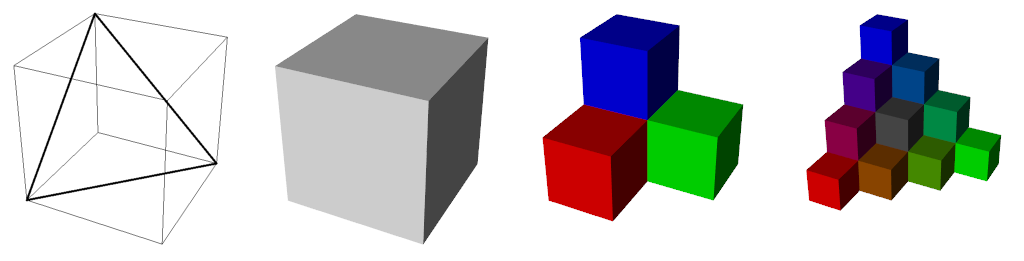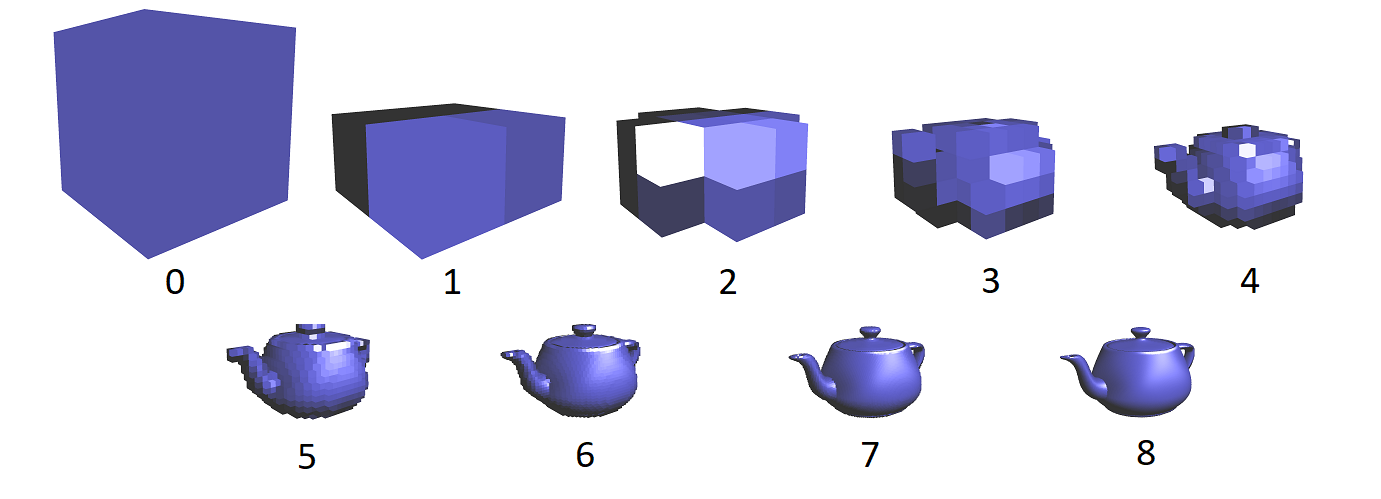# Structured Point Clouds (SPCs)¶

Structured Point Clouds (SPC) is a sparse octree-based representation that is useful to organize and compress 3D geometrically sparse information. They are also known as sparse voxelgrids, quantized point clouds, and voxelized point clouds.Kaolin supports a number of operations to work with SPCs, including efficient ray-tracing and convolutions.

The SPC data structure is very general. In the SPC data structure, octrees provide a way to store and efficiently retrieve coordinates of points at different levels of the octree hierarchy. It is also possible to associate features to these coordinates using point ordering in memory. Below we detail the low-level representations that comprise SPCs and allow corresponding efficient operations. We also provide a convenience container for these low-level attributes.

Some of the conventions are also defined in Neural Geometric Level of Detail: Real-time Rendering with Implicit 3D Surfaces which uses SPC as an internal representation.

Warning

Structured Point Clouds internal layout and structure is still experimental and may be modified in the future.

## Octree¶

Core to SPC is the octree, a tree data structure where each node have up to 8 childrens. We use this structure to do a recursive three-dimensional space partitioning, i.e: each node represents a partitioning of its 3D space (partition) of $$(2, 2, 2)$$. The octree then contains the information necessary to find the sparse coordinates.

In SPC, a batch of octrees is represented as a tensor of bytes. Each bit in the byte array octrees represents the binary occupancy of an octree bit sorted in Morton Order. The Morton order is a type of space-filling curve which gives a deterministic ordering of integer coordinates on a 3D grid. That is, for a given non-negative 1D integer coordinate, there exists a bijective mapping to 3D integer coordinates.

Since a byte is a collection of 8 bits, a single byte octrees[i] represents an octree node where each bit indicate the binary occupancy of a child node / partition as depicted below:For each octree, the nodes / bytes are following breadth-first-search order (with Morton order for childrens order), and the octree bytes are then Packed to form octrees. This ordering allows efficient tree access without having to explicilty store indirection pointers.

The binary occupancy values in the bits of octrees implicitly encode position data due to the bijective mapping from Morton codes to 3D integer coordinates. However, to provide users a more straight forward interface to work with these octrees, SPC provides auxilary information such as points which is a Packed tensor of 3D coordinates. Refer to the Related attributes section for more details.

Currently SPCs are primarily used to represent 3D surfaces, and so all the leaves are at the same level (depth). This allow very efficient processing on GPU, with custom CUDA kernels, for ray-tracing and convolution.

The structure contains finer details as you go deeper in to the tree. Below are the Levels 0 through 8 of a SPC teapot model:The nodes of the octrees can contain information beyond just the 3D coordinates of the nodes, such as RGB color, normals, feature maps, or even differentiable activation maps processed by a convolution.

We follow a Structure of Arrays approach to store additional data for maximum user extensibility. Currently the features would be tensors of shape $$(\text{num_nodes}, \text{feature_dim})$$ with num_nodes being the number of nodes at a specific level of the octrees, and feature_dim the dimension of the feature set (for instance 3 for RGB color). Users can freely define their own feature data to be stored alongside SPC.

## Conversions¶

Structured point clouds can be derived from multiple sources.

We can construct octrees from unstructured point cloud data, from sparse voxelgrids or from the level set of an implicit function $$f(x, y, z)$$.

## Convolutions¶

We provide several sparse convolution layers for structured point clouds. Convolutions are characterized by the size of the input and output channels, an array of kernel_vectors, and possibly the number of levels to jump, i.e., the difference in input and output levels.

An example of how to create a $$3 \times 3 \times 3$$ kernel follows:

>>> vectors = []
>>> for i in range(-1, 2):
>>>     for j in range(-1, 2):
>>>         for k in range(-1, 2):
>>>             vectors.append([i, j, k])
>>> Kvec = torch.tensor(vectors, dtype=torch.short, device=device)
>>> Kvec
tensor([[-1, -1, -1],
[-1, -1,  0],
[-1, -1,  1],
...
...
[ 1,  1, -1],
[ 1,  1,  0],
[ 1,  1,  1]], device='cuda:0', dtype=torch.int16)


The kernel vectors determine the shape of the convolution kernel. Each kernel vector is added to the position of a point to determine the coordinates of points whose corresponding input data is needed for the operation. We formalize this notion using the following neighbor function:

$n(i,k) = \text{ID}\left(P_i+\overrightarrow{K}_k\right)$

that returns the index of the point within the same level found by adding kernel vector $$\overrightarrow{K}_k$$ to point $$P_i$$. Given the sparse nature of SPC data, it may be the case that no such point exists. In such cases, $$n(i,k)$$ will return an invalid value, and data accesses will be treated like zero padding.

Transposed convolutions are defined by the transposed neighbor function

$n^T(i,k) = \text{ID}\left(P_i-\overrightarrow{K}_k\right)$

The value jump is used to indicate the difference in levels between the iput features and the output features. For convolutions, this is the number of levels to downsample; while for transposed convolutions, jump is the number of levels to upsample. The value of jump must be positive, and may not go beyond the highest level of the octree.

### Examples¶

You can create octrees from sparse feature_grids (of shape $$(\text{batch_size}, \text{feature_dim}, \text{height}, \text{width}, \text{depth})$$):

>>> octrees, lengths, features = kaolin.ops.spc.feature_grids_to_spc(features_grids)


or from point cloud (of shape $$(\text{num_points, 3})$$):

>>> qpc = kaolin.ops.spc.quantize_points(pc, level)
>>> octree = kaolin.ops.spc.unbatched_points_to_octree(qpc, level)


To use convolution, you can use the functional or the torch.nn.Module version like torch.nn.functional.conv3d and torch.nn.Conv3d:

>>> max_level, pyramids, exsum = kaolin.ops.spc.scan_octrees(octrees, lengths)
>>> point_hierarchies = kaolin.ops.spc.generate_points(octrees, pyramids, exsum)
>>> kernel_vectors = torch.tensor([[0, 0, 0], [0, 0, 1], [0, 1, 0], [0, 1, 1],
[1, 0, 0], [1, 0, 1], [1, 1, 0], [1, 1, 1]],
dtype=torch.ShortTensor, device='cuda')
>>> conv = kaolin.ops.spc.Conv3d(in_channels, out_channels, kernel_vectors, jump=1, bias=True).cuda()
>>> # With functional
>>> out_features, out_level = kaolin.ops.spc.conv3d(octrees, point_hierarchies, level, pyramids,
...                                                 exsum, coalescent_features, weight,
...                                                 kernel_vectors, jump, bias)
>>> # With nn.Module and container class
>>> input_spc = kaolin.rep.Spc(octrees, lengths)
>>> conv
>>> out_features, out_level = kaolin.ops.spc.conv_transpose3d(
...     **input_spc.to_dict(), input=out_features, level=level,
...     weight=weight, kernel_vectors=kernel_vectors, jump=jump, bias=bias)


To apply ray tracing we currently only support non-batched version, for instance here with RGB values as per point features:

>>> max_level, pyramids, exsum = kaolin.ops.spc.scan_octrees(
...     octree, torch.tensor([len(octree)], dtype=torch.int32, device='cuda')
>>> point_hierarchy = kaolin.ops.spc.generate_points(octrees, pyramids, exsum)
>>> ridx, pidx, depth = kaolin.render.spc.unbatched_raytrace(octree, point_hierarchy, pyramids, exsum,
...                                                          origin, direction, max_level)
>>> first_hits_rgb = rgb[first_hits_point - pyramids[max_level - 2]]


## Going further with SPC:¶

### Examples:¶

See our Jupyter notebook for a walk-through of SPC features:

examples/tutorial/understanding_spcs_tutorial.ipynb

And also our recipes for simple examples of how to use SPC:

### SPC Documentation:¶

Functions useful for working with SPCs are available in the following modules: